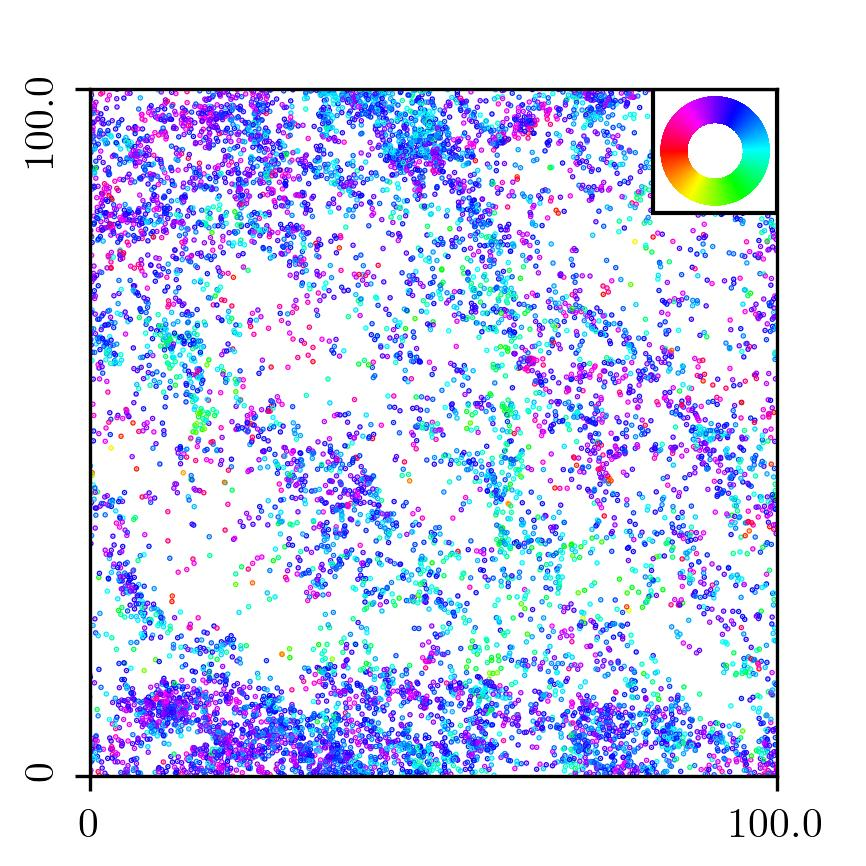## AAPPP

AAPPP is an open source python package written in C that can be used to simulate the 2-d Vicsek model and many more 2-d models of aligning active particles.

You can find it at https://github.com/kuersten/aappp/.

### Installation

Before installation you need python3 and pip. Installation should work on Linux and Mac as follows

``````
bash install.bash
```
```

### Simulations

You can run simulations from within python3. To initialize a simulation just type

``````
import aappp
mysim=aappp.aappp_init(Lx=100, Ly=100, v=1, R=1, eta=0.3, N=10000, seed=1)
```
```

Now you are ready to perform some Vicsek time steps

``````
aappp.VM_update_timesteps(mysim, 10000)
```
```

You can save the final simulation state for later use and free memory

``````
aappp.aappp_save(mysim, 'mydata.dat')
aappp.aappp_free(mysim)
```
```

You can analyze or plot the simulation results yourself, however, an easy way to plot a snapshot is just using the example script 'aappp_snapshot.py'

In terminal you can just type

``````
python3 aappp_snapshot.py -f mydata.dat -w
```
```Each point corresponds to the position of a particle. The color encodes the direction of motion.

``````